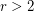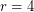# r-regular graphs are not uniquely hamiltonian.

 Importance: High ✭✭✭
 Author(s): Sheehan, John
 Subject: Graph Theory » Basic Graph Theory » » Cycles
 Keywords: hamiltonian regular uniquely hamiltonian
 Posted by: Robert Samal on: July 24th, 2007
Conjecture   Ifis a finite-regular graph, where, thenis not uniquely hamiltonian.

(Reproduced from [M].)

A graphis said to be uniquely hamiltonian if it contains precisely one Hamiltonian cycle.

This conjecture has been proved for all odd values of[T] and for all even values of[H]. By Petersen's theorem, it would suffice to prove it for.

## Bibliography

[H] P. Haxell, Oberwolfach reports, 2006.

[M] Bojan Mohar, Problem of the Month

*[S] John Sheehan: The multiplicity of Hamiltonian circuits in a graph. Recent advances in graph theory (Proc. Second Czechoslovak Sympos., Prague, 1974), pp. 477-480. Academia, Prague, 1975, MathSciNet

[T] A.G. Thomason, Hamiltonian cycles and uniquely edge colourable graphs. Advances in graph theory (Cambridge Combinatorial Conf., Trinity College, Cambridge, 1977). Ann. Discrete Math. 3 (1978), Exp. No. 13, 3 pp.

* indicates original appearance(s) of problem.